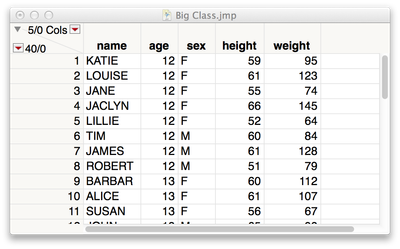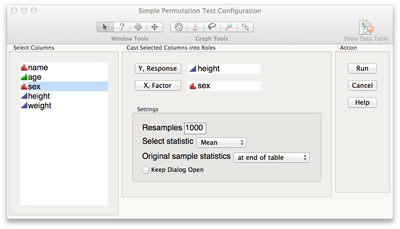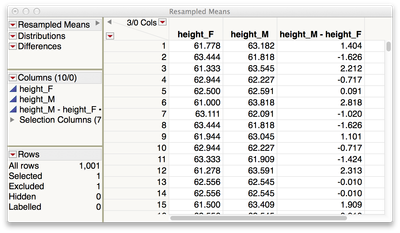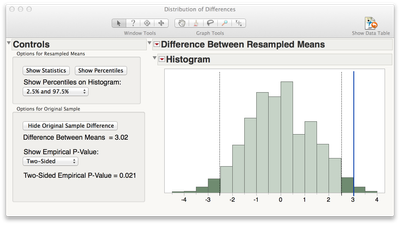## Permutation Test for Two Means or Medians

Use this simple permutation test add-in to illustrate the concept of a p-value for a test for involving the difference between two means or two medians.  The add-in produces a table of resample means (or medians), with saved scripts for exploring these values and for comparing the original statistic to the resample statistics.

Use Notes:

The add-in will use the active JMP data table, or will prompt you to open a data table.  Here, we use the Big Class data from the sample data directory in JMP.  Note that the data must be stacked (i.e. the Y values must be stacked in one column, and the X labels must be stacked in a separate column).Notes on the Dialog Window:

• Select a numeric (continuous) response
• Select a categorical (nominal or ordinal) factor - if the factor has more than 2 levels you will be asked to select the two levels of interest
• Specify the number of resamples to draw - the default is 1000, and this is very quick
• Specify the statistic to resample (the mean or the median)
• Specify where to place the original sample statistics (this is for comparison)
• Click the Help button for additional informationHow it works, and the results:

For each resample, the labels for the factor are shuffled and the means for the two levels are computed. The resulting resample table has two columns of resample values for the specified number of resamples (plus the original statistics), a column of differences between these values, and some saved scripts.How to use the results:

To manually compare the original statistics to the differences between the resample values, right-click on the difference column and select Sort. Then, count the number of differences that are more extreme than the original statistics, and convert this to a percentile.

Example:  If 10 of the resample differences are as extreme or more extreme than the observed difference, then the empirical p-value is 10/1000 = 0.010.

To graphically compare the original statistic to the differences between the resample values, run the Differences script (in the top left corner of the resample table).  This produces a histogram of the differences between the resampled values, with options to:

• Explore percentiles of this distribution,
• Compare the observed difference to the distribution of differences, and
• Calculate an empirical p-value.

In this example, the observed difference between means is 3.02.  The two-sided empirical p-value is 0.021.  This means that only 21 of the 1000 differences were as extreme or more extreme than our observed difference of 3.02.Interpretation?  The null hypothesis is that the means for the two groups (heights for males and females, in this case) are equal.  If the means were truly equal, it is unlikely that we would observe a difference in means as or more extreme than 3.02 by chance alone.  Just how unlikely is this?  It happened only 0.021, or 2.1% of the time!

Credits:

The code behind this permutation test was originally developed by Laura Schultz of Rowan University.  Thanks to Laura for sharing this with us!  The code was further developed and additional features were added by julian (Julian Parris, JMP Academic Ambassador).  Thanks Julian!

Comments, feedback or suggestions, please let us know!  Do you have a cool JMP-based simulator or teaching tool that you'd be willing to share with other stat educators?  Please consider posting in the JMP Academic .